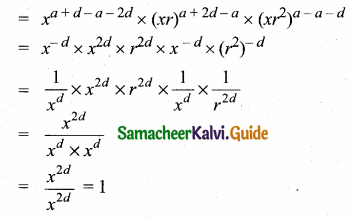Students can download Maths Chapter 2 Numbers and Sequences Ex 2.7 Questions and Answers, Notes, Samacheer Kalvi 10th Maths Guide Pdf helps you to revise the complete Tamilnadu State Board New Syllabus, helps students complete homework assignments and to score high marks in board exams.

Tamilnadu Samacheer Kalvi 10th Maths Solutions Chapter 2 Numbers and Sequences Ex 2.7

Question 1.
Which of the following sequences are in G.P?
(i) 3,9,27,81,…
(ii) 4,44,444,4444,…
(iii) 0.5,0.05,0.005,
(iv) $$\frac { 1 }{ 3 }$$,$$\frac { 1 }{ 6 }$$,$$\frac { 1 }{ 12 }$$, ………….
(v) 1, -5, 25,-125,…
(vi) 120, 60, 30, 18,…
(vii) 16, 4, 1, $$\frac { 1 }{ 4 }$$, ……….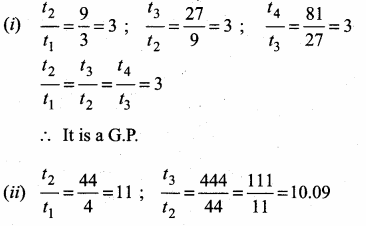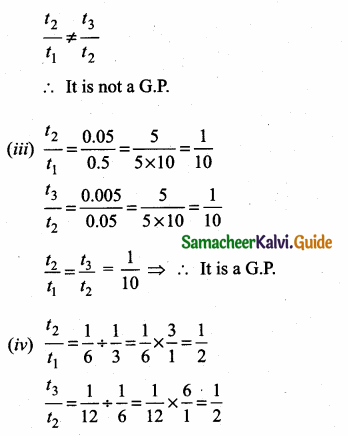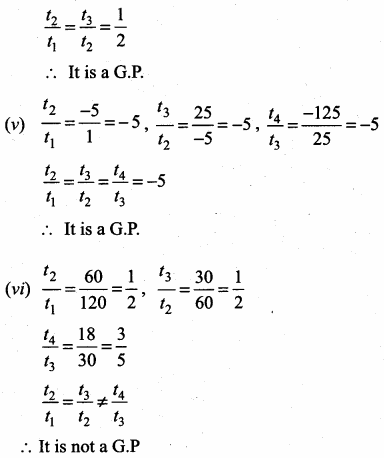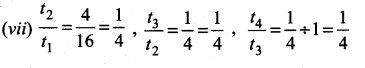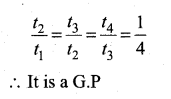Question 2.
Write the first three terms of the G.P. whose first term and the common ratio are given below.
(i) a = 6, r = 3
a = 6, r = 3
ar = 6 × 3 = 18,
ar2 = 6 × 9 = 54
The three terms are 6, 18 and 54

(ii) a = $$\sqrt { 2 }$$, r = $$\sqrt { 2 }$$.
ar = $$\sqrt { 2 }$$ × $$\sqrt { 2 }$$ = 2,
ar2 = $$\sqrt { 2 }$$ × 2 = 2 $$\sqrt { 2 }$$
The three terms are $$\sqrt { 2 }$$, 2 and 2$$\sqrt { 2 }$$

(iii) a = 1000, r = $$\frac { 2 }{ 5 }$$
ar = 1000 × $$\frac { 2 }{ 5 }$$ = 400,
ar2 = 1000 × $$\frac { 4 }{ 25 }$$ = 40 × 4 = 160
The three terms are 1000,400 and 160.

Question 3.
In a G.P. 729, 243, 81,… find t7.
The G.P. is 729, 243, 81,….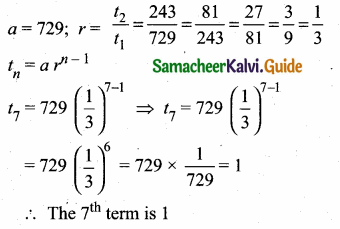Question 4.
Find x so that x + 6, x + 12 and x + 15 are consecutive terms of a Geometric Progression
Solution:
G.P = x + 6, x + 12, x + 15
In G.P r = $$\frac{t_{2}}{t_{1}}=\frac{t_{3}}{t_{2}}$$
$$\frac{x+12}{x+6}=\frac{x+15}{x+12}$$
(x + 12)2 = (x + 6) (x + 5)
x2 + 24x + 144 = x2 + 6x + 15x + 90
24x – 21x = 90 – 144
3x = -54
x = $$\frac { -54 }{ 3 }$$ = -18
x = -18Question 5.
Find the number of terms in the following G.P.

(i) 4,8,16,…,8192?
Here a = 4; r = $$\frac { 8 }{ 4 }$$ = 2
tn = 8192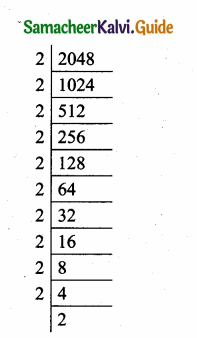a . rn-1 = 8192 ⇒ 4 × 2n-1 = 8192
2n-1 = $$\frac { 8192 }{ 4 }$$ = 2048
2n-1 = 211 ⇒ n – 1 = 11
n = 11 + 1 ⇒ n = 12
Number of terms = 12

(ii) $$\frac { 1 }{ 3 }$$, $$\frac { 1 }{ 9 }$$, $$\frac { 1 }{ 27 }$$, ……………, $$\frac { 1 }{ 2187 }$$
a = $$\frac { 1 }{ 3 }$$ ; r = $$\frac { 1 }{ 9 }$$ ÷ $$\frac { 1 }{ 3 }$$ = $$\frac { 1 }{ 9 }$$ × $$\frac { 3 }{ 1 }$$ = $$\frac { 1 }{ 3 }$$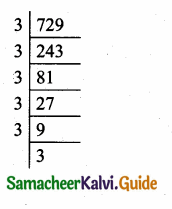tn = $$\frac { 1 }{ 2187 }$$
a. rn-1 = $$\frac { 1 }{ 2187 }$$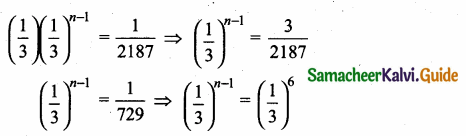n – 1 = 6 ⇒ n = 6 + 1 = 7
Number of terms = 7Question 6.
In a G.P. the 9th term is 32805 and 6th term is 1215. Find the 12th term.
Given, 9th term = 32805
a. rn-1 = $$\frac { 1 }{ 2187 }$$
t9 = 32805 [tn = arn-1]
a.r8 = 32805 …..(1)
6th term = 1215
a.r5 = 1215 …..(2)
Divide (1) by (2)
$$\frac{a r^{8}}{a r^{5}}$$ = $$\frac { 32805 }{ 1215 }$$ ⇒ r3 = $$\frac { 6561 }{ 243 }$$
= $$\frac { 2187 }{ 81 }$$ = $$\frac { 729 }{ 27 }$$ = $$\frac { 243 }{ 9 }$$ = $$\frac { 81 }{ 3 }$$
r3 = 27 ⇒ r3 = 33
r = 3
Substitute the value of r = 3 in (2)
a. 35 = 1215
a × 243 = 1215
a = $$\frac { 1215 }{ 243 }$$ = 5
Here a = 5, r = 3, n = 12
t12 = 5 × 3(12-1)
= 5 × 311
∴ 12th term of a G.P. = 5 × 311Question 7.
Find the 10th term of a G.P. whose 8th term is 768 and the common ratio is 2.
Solution:
t8 = 768 = ar7
r = 2
t10 = ar9 = ar7 × r × r
= 768 × 2 × 2 = 3072Question 8.
If a, b, c are in A.P. then show that 3a, 3b, 3c are in G.P.
a, b, c are in A.P.
t2 – t1 = t3 – t2
b – a = c – b
2b = a + c …..(1)
3a, 3b, 3c are in G.P.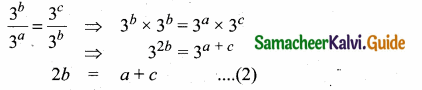From (1) and (2) we get
3a, 3b, 3c are in G.P.

Question 9.
In a G.P. the product of three consecutive terms is 27 and the sum of the product of two terms taken at a time is $$\frac { 57 }{ 2 }$$. Find the three terms.
Let the three terms of the G.P. be $$\frac { a }{ r }$$, a, ar
Product of three terms = 27
$$\frac { a }{ r }$$ × a × ar = 27
a3 = 27 ⇒ a3 = 33
a = 3
Sum of the product of two terms taken at a time is $$\frac { 57 }{ 2 }$$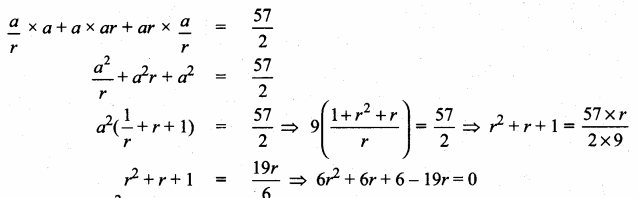6r2 – 13r + 6 = 0
6r2 – 9r – 4r + 6 = 0
3r (2r – 3) -2(2r – 3) = 0
(2r – 3) (3r – 2) = 0
2r – 3 = 0 or 3r – 2 = 0
2r = 3 (or) 3r – 2 = 0
r = $$\frac { 3 }{ 2 }$$ (or) r = $$\frac { 2 }{ 3 }$$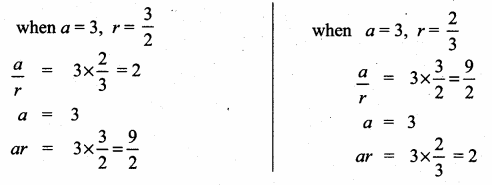∴ The three terms are 2, 3 and $$\frac { 9 }{ 2 }$$ or $$\frac { 9 }{ 2 }$$, 3 and 2Question 10.
A man joined a company as Assistant Manager. The company gave him a starting salary of ₹60,000 and agreed to increase his salary 5% annually. What will be his salary after 5 years?
Starting salary (a) = ₹ 60000
Increased salary = 5% of starting salary
= $$\frac { 5 }{ 100 }$$ × 60000
= ₹ 3000
Starting salary for the 2nd year = 60000 + 3000
= ₹ 63000
Year increase = 5% of 63000
= $$\frac { 5 }{ 100 }$$ × 63000
= ₹ 3150
Starting salary for the 3rd year = 63000 + 3150
= ₹ 66150
60000, 63000, 66150,…. form a G.P.
a = 60000; r = $$\frac { 63000 }{ 60000 }$$ = $$\frac { 63 }{ 60 }$$ = $$\frac { 21 }{ 20 }$$
tn = ann-1
t5 = (60000) ($$\frac { 21 }{ 20 }$$)4
= 60000 × $$\frac { 21 }{ 20 }$$ × $$\frac { 21 }{ 20 }$$ × $$\frac { 21 }{ 20 }$$ × $$\frac { 21 }{ 20 }$$
= $$\frac{6 \times 21 \times 21 \times 21 \times 21}{2 \times 2 \times 2 \times 2}$$
= 72930.38
5% increase = $$\frac { 5 }{ 100 }$$ × 72930.38
= ₹ 3646.51
Salary after 5 years = ₹ 72930.38 + 3646.51
= ₹ 76576.90
= ₹ 76577Question 11.
Sivamani is attending an interview for a job and the company gave two offers to him. Offer A: ₹20,000 to start with followed by a guaranteed annual increase of 6% for the first 5 years.
Offer B: ₹22,000 to start with followed by a guaranteed annual increase of 3% for the first 5 years.
What is his salary in the 4th year with respect to the offers A and B?
Starting salary (a) = ₹ 20,000
Annual increase = 6% of 20000
= $$\frac { 5 }{ 100 }$$ × 20000
= ₹ 1200
Salary for the 2nd year = ₹ 20000 + 1200
= ₹ 21200
Here a = 20,000; r = $$\frac { 21200 }{ 20000 }$$ = $$\frac { 212 }{ 200 }$$ = $$\frac { 106 }{ 100 }$$ = $$\frac { 53 }{ 50 }$$
n = 4 years
tn = arn-1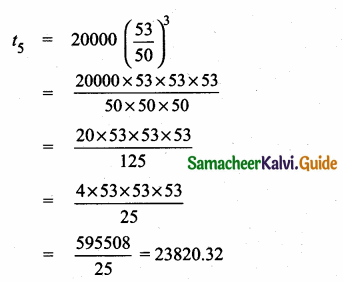Salary at the end of 4th year = 23820

For B
Starting salary = ₹ 22000
(a) = 22000
Annual increase = 3% of 22000
= $$\frac { 3 }{ 100 }$$ × 22000
= ₹ 660
Salary for the 2nd year = ₹ 22000 + ₹ 660
= ₹ 22,660
Here a = 22000; r = $$\frac { 22660 }{ 22000 }$$
= $$\frac { 2266 }{ 2200 }$$ = $$\frac { 1133 }{ 1100 }$$ = $$\frac { 103 }{ 100 }$$
Salary at the end of 4th year = 22000 × ($$\frac { 103 }{ 100 }$$)4-1
= 22000 × ($$\frac { 103 }{ 100 }$$)3
= 22000 × $$\frac { 103 }{ 100 }$$ × $$\frac { 103 }{ 100 }$$ × $$\frac { 103 }{ 100 }$$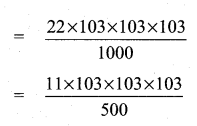= 24039.99 = 24040
4th year Salary for A = ₹ 23820 and 4th year Salary for B = ₹ 24040Question 12.
If a, b, c are three consecutive terms of an A.P. and x, y, z are three consecutive terms of a G.P. then prove that xb-c × yc-a × za-b = 1